### Home > MC2 > Chapter 5 > Lesson 5.2.3 > Problem5-67

5-67.

Write an algebraic expression for each mat below. Then use the legal moves that you have developed to simplify each mat and, if possible, decide which expression is greater. Homework Help ✎

 a.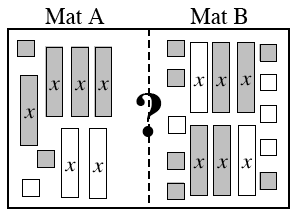b.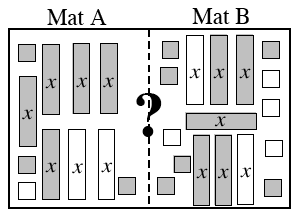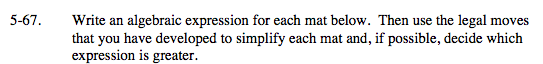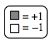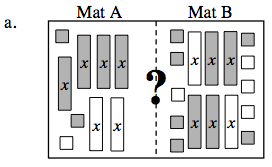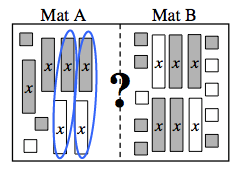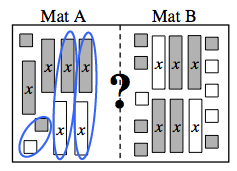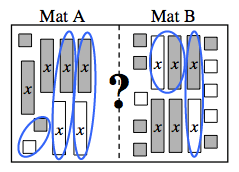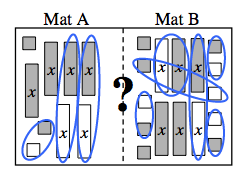Mat A

How many zero pairs of x-tiles are there?

There are two zero pairs of x-tiles. Now how many zero pairs of unit tiles are there?

There is one pair of zero unit tiles. Now remove the zero pairs and what is left?

2x+1

Mat B

How many zero pairs of x-tiles are there?

There are two zero pairs of x-tiles. Now how many zero pairs of unit tiles are there?

There are four pairs of zero unit tiles. Now remove the zero pairs and what is left?

2x+2
Now remove anything that is the same on both sides. Which side is greater?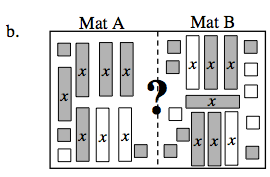Mat A

3x+2

Mat B

3x+2
Now remove 3x from each side because they are the same.
2=2; They are equal.